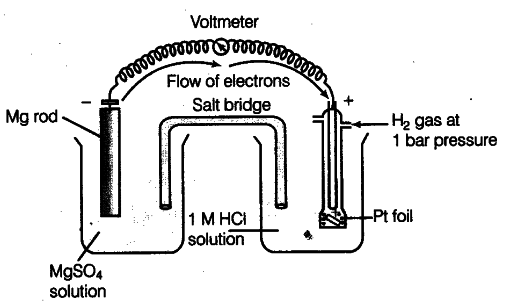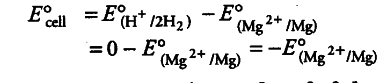# How would you determine the standard electrode potential of the system \${{Mg}^{2+}}\$/Mg?

E° value of {{Mg}^{2+}}/Mg electrode is determined by setting up an electrochemical cell. For this purpose, a Mg electrode is dipped in 1 M MgSO_{4} solution which acts as one half-cell, i.e. oxidation half-cell.In the same way, the standard hydrogen electrode acts as the other half-cell, i.e. reduction half-cell. The deflection of voltmeter placed in the cell circuit is towards the Mg electrode which indicates the direction of flow of current. The cell may be represented as:
Mg | {{Mg}^{2+}} (1 M) | {{H}^{+}}(1M)| H_{2}(l bar), Pt (s)
The reading as given by voltmeter, gives E° cell